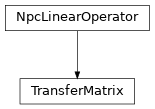# TransferMatrix¶

Inheritance DiagramMethods

 TransferMatrix.__init__(bra, ket[, …]) Initialize self. Return the hermitian conjugate of self TransferMatrix.eigenvectors([num_ev, …]) Find (dominant) eigenvector(s) of self using scipy.sparse. Return a diagonal matrix as initial guess for the eigenvector. Given vec as an npc.Array, apply the transfer matrix. Contract self to a matrix.

Class Attributes and Properties

 TransferMatrix.acts_on
class tenpy.networks.mps.TransferMatrix(bra, ket, shift_bra=0, shift_ket=None, transpose=False, charge_sector=0, form='B')[source]

Transfer matrix of two MPS (bra & ket).

For an iMPS in the thermodynamic limit, we often need to find the ‘dominant RP’ (and LP). This mean nothing else than to take the transfer matrix of the unit cell and find the (right/left) eigenvector with the largest (magnitude) eigenvalue, since it will dominate $$(TM)^n RP$$ (or $$LP (TM)^n$$) in the limit $$n \rightarrow \infty$$ - whatever the initial RP is. This class provides exactly that functionality with eigenvectors().

Given two MPS, we define the transfer matrix as:

|    ---M[i]---M[i+1]- ... --M[i+L]---
|       |      |             |
|    ---N[j]*--N[j+1]* ... --N[j+L]*--


Here the M denotes the matrices of the bra and N the ones of the ket, respectively. To view it as a matrix, we combine the left and right indices to pipes:

|  (vL.vL*) ->-TM->- (vR.vR*)   acting on  (vL.vL*) ->-RP


Note that we keep all M and N as copies.

Deprecated since version 0.6.0: The default for shift_ket was the value of shift_bra, this will be changed to 0.

Parameters
• bra (MPS) – The MPS which is to be (complex) conjugated.

• ket (MPS) – The MPS which is not (complex) conjugated.

• shift_bra (int) – We start the N of the bra at site shift_bra (i.e. the j in the above network).

• shift_ket (int | None) – We start the M of the ket at site shift_ket (i.e. the i in the above network). None is deprecated, default will be changed to 0 in the future.

• transpose (bool) – Wheter self.matvec acts on RP (False) or LP (True).

• charge_sector (None | charges | 0) – Selects the charge sector of the vector onto which the Linear operator acts. None stands for all sectors, 0 stands for the zero-charge sector. Defaults to 0, i.e., assumes the dominant eigenvector is in charge sector 0.

• form ('B' | 'A' | 'C' | 'G' | 'Th' | None | tuple(float, float)) – In which canonical form we take the M and N matrices.

L

Number of physical sites involved in the transfer matrix, i.e. the least common multiple of bra.L and ket.L.

Type

int

shift_bra

We start the N of the bra at site shift_bra.

Type

int

shift_ket

We start the M of the ket at site shift_ket. None defaults to shift_bra.

Type

int | None

transpose

Wheter self.matvec acts on RP (True) or LP (False).

Type

bool

qtotal

Total charge of the transfer matrix (which is gauged away in matvec).

Type

charges

form

In which canonical form (all of) the M and N matrices are.

Type

tuple(float, float) | None

flat_linop

Class lifting matvec() to ndarrays in order to use speigs().

Type

FlatLinearOperator

pipe

Pipe corresponding to '(vL.vL*)' for transpose=False or to '(vR.vR*)' for transpose=True.

Type

LegPipe

label_split

['vL', 'vL*'] if tranpose=False or ['vR', 'vR*'] if transpose=True.

_bra_N

Complex conjugated matrices of the bra, transposed for fast matvec.

Type

list of npc.Array

_ket_M

The matrices of the ket, transposed for fast matvec.

Type

list of npc.Array

matvec(vec)[source]

Given vec as an npc.Array, apply the transfer matrix.

Parameters

vec (Array) – Vector to act on with the transfermatrix. If not transposed, vec is the right part RP of an environment, with legs '(vL.vL*)' in a pipe or splitted. If transposed, the left part LP of an environment with legs '(vR*.vR)'.

Returns

mat_vec – The tranfer matrix acted on vec, in the same form as given.

Return type

Array

initial_guess(diag=1.0)[source]

Return a diagonal matrix as initial guess for the eigenvector.

Parameters

diag (float | 1D ndarray) – Should be 1. for the identity or some singular values squared.

Returns

mat – A 2D array with diag on the diagonal such that matvec() can act on it.

Return type

Array

eigenvectors(num_ev=1, max_num_ev=None, max_tol=1e-12, which='LM', v0=None, **kwargs)[source]

Find (dominant) eigenvector(s) of self using scipy.sparse.

If no charge_sector was selected, we look in all charge sectors.

Parameters
• num_ev (int) – Number of eigenvalues/vectors to look for.

• max_num_ev (int) – scipy.sparse.linalg.speigs() somtimes raises a NoConvergenceError for small num_ev, which might be avoided by increasing num_ev. As a work-around, we try it again in the case of an error, just with larger num_ev up to max_num_ev. None defaults to num_ev + 2.

• max_tol (float) – After the first NoConvergenceError we increase the tol argument to that value.

• which (str) – Which eigenvalues to look for, see scipy.sparse.linalg.speigs.

• **kwargs – Further keyword arguments given to speigs().

Returns

• eta (1D ndarray) – The eigenvalues, sorted according to which.

• w (list of Array) – The eigenvectors corresponding to eta, as npc.Array with LegPipe.

adjoint()[source]

Return the hermitian conjugate of self

If self is hermitian, subclasses can choose to implement this to define the adjoint operator of self.

to_matrix()[source]

Contract self to a matrix.

If self represents an operator with very small shape, e.g. because the MPS bond dimension is very small, an algorithm might choose to contract self to a single tensor.

Returns

matrix – Contraction of the represented operator.

Return type

Array